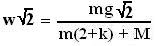## Monday, August 13, 2007

### Irodov Problem 1.78Let the tension in the string me T. Let the acceleration of mass m in the downwards direction be w. AB, CD and EF are three different sections of the string. Thus the total length AB + CD + EF must be conserved. If the mass m moves down by x units, the length of AB increases by x units. The length CD remains the same since the moving pulleys move only in the horizontal direction at the same rate. For the total length to be conserved, thus, EF must decrease by x units. This means that the pulley and hence the mass M accelerates at the same rate w in the horizontal direction. Since the mass M accelerates in the horizontal direction, it pushes mass m
along with it and this results in a normal reaction N between M and m (as shown in the figure). The friction force between M and m is then equal to Nk.

Forces acting on mass m: The forces acting on mass are depicted in the figure. In the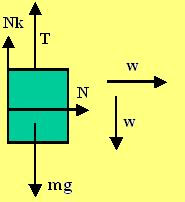horizontal direction there is only one force acting on the mass - the normal reaction from mass M pushing it. The mass m accelerates
along the horizontal direction with an acceleration w. Thus, we have

N = mw (1)

In the vertical direction there are three forces acting on the mass, i) the tension in the string T pulling it up ii) the force of friction Nk opposing the downward motion of the mass and iii) the force of gravity mg pulling it down. The mass accelerates downwards with an acceleration w. Thus, we have,

mg - T - Nk = mw (2)

From (1) and (2) we have,

mg - T = mw(1+k) (3)

Forces acting on mass M: The forces acting on M are depicted in the figure. We shall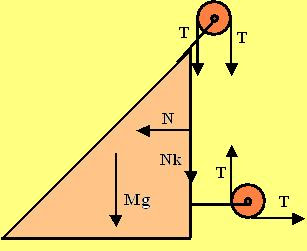consider only the forces acting in the horizontal direction for this problem. There are two forces acting in the horizontal direction, i) the normal reaction from m pushing it back and ii) the tension in the string T on the lower pulley pulling it
forward. The mass M accelerates at a rate w. Thus, we have,

T - N = Mw (4)

From (4) and (1) we have,

T = (M+m)w (5)

From (5) and (3)

mg - (M+m)w = mw(1+k) or,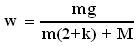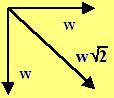The mass m accelerates both in the horizontal and vertical direction at an acceleration w. Thus, the net acceleration vector is given by,